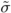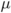# Volatility arbitrage, some results

As promised, here is some of the mathematics behind hedging options when you think that there is an arbitrage opportunity.

Let’s keep the problem simple. You are in a Black-Scholes world. Volatility is constant. But the market is pricing an option using the wrong volatility, implied volatility is lower than actual volatility, so the option is cheap. You buy the option, but then to make money you must hedge away market risk using the underlying. Do you use a delta based on the actual volatility or on implied volatility?

Case 1: Hedge using actual volatility

If you use actual volatility then you make a guaranteed profit, whose present value is the difference between Black-Scholes using actual volatility and Black-Scholes using implied:whereis actual volatility andis implied. This is the guaranteed profit, regardless of how the stock behaves, it is path independent. Unfortunately, you get wild P&L swings hedging this way.

Case 2: Hedge using implied volatility

It is more common to hedge using implied volatility. Now the profit is path dependent, but at least there aren’t the wild swings from day to day. Each time step you make a profit ofThis is always positive (as long as actual volatility is greater than implied) but the total by expiration depends on how the stock behaves.

The expected profit is thenThis depends on the growth rate of the stock. Note that this is the expected profit. Although you won’t lose money hedging this way you will not know a priori how much profit you will make.

So would you rather have a known profit but with daily P&L swings, or a positive daily P&L but random final profit? Perhaps the deciding factor is that when you hedge with implied volatility you will make a profit whenever you are on the right side of the trade (buy when implied vol is lower than actual and sell when it is higher). You can see this from the above formula for the profit each time step.

All of the details are contained in Ahmad & Wilmott 2005 “Which free lunch would you like today, Sir?” Wilmottmagazine, November issue.

P